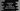# JavaScript program to check if a number is power of another number## JavaScript program to check if a number is power of another number:

In this post, we will learn how to check if a number is power of another number or not. For example, if the first number is 27 and the second number is 3, then it will be a success because 3 ^ 3 = 27.

We need to use a loop to solve this problem. Inside the loop, we can check for each numbers starting 0 , if the power of the second number to this number is equal to the first number.

We can use a for loop or while loop to solve it. Both will use the same steps. Let’s check the algorithm first before writing the program.

### Algorithm:

We will use the below algorithm to solve this problem:

• Suppose, firstNumber is the bigger number and secondNumber is the smaller number
• Start one loop to run from 0 to firstNumber. In each iteration of the loop, check if the power of secondNumber to current iterating value is equal to firstNumber or not.
• If yes, return true. If not, move to the next iteration.
• If the power value is greater than firstNumber, we don’t have to check for other numbers as it is already more than firstNumber. So, return false.
• After the loop ends, return false.

### By using a for loop:

Let’s try to fix this by using a for loop. Below is the complete program:

``````const isPowerOf = (firstNumber, secondNumber) => {

for (let i = 0; i < firstNumber; i++) {
let pow = Math.pow(secondNumber, i);
if (pow === firstNumber) {
return true;
}
if (pow > firstNumber) {
return false;
}
}

return false;
}

let firstNumber = 27;
let secondNumber = 3;

console.log(isPowerOf(firstNumber, secondNumber));``````

Here,

• isPowerOf method is used to check if firstNumber is power of secondNumber.
• Inside this method, we are running a for loop. This loop runs from 0 to firstNumber - 1. For each value, it is calculating the power of secondNumber to that value. We are using Math.pow to find the power.
• If the value of power is equal to the firstNumber, it returns true. If it is more than firstNumber, it returns false.
• Once the loop ends, it returns false.

If you run this program, it will print true.

### By using a while loop:

We can also solve it by using a while loop. Below program does that:

``````const isPowerOf = (firstNumber, secondNumber) => {

let i = 0;
while (i < firstNumber) {
let pow = Math.pow(secondNumber, i);

if (pow === firstNumber) {
return true;
}
if (pow > firstNumber) {
return false;
}
i++;
}

return false;
}

let firstNumber = 59049;
let secondNumber = 3;

console.log(isPowerOf(firstNumber, secondNumber));``````

It is similar to the for loop approach. The only difference is that we have initialized i before while starts. We are incrementing its value at the end of the loop. Other things are similar to the above program.

If you run this program, it will print true.